TitleIntermediate Algebra
Answer/Discussion to Practice Problems
Tutorial 7: Linear Equations in One VariableAnswer/Discussion to 1a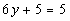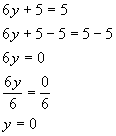*Inverse of add. 5 is sub 5 from both sides   *Inverse of mult. by 6 is div. both sides by 6

 If you put 0 back in for y in the original problem you will see that 0 is the solution we are looking for. (return to problem 1a)Answer/Discussion to 1b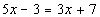*Get all x terms on one side   *Inverse of sub. 3 is add. 3 to both sides   *Inverse of mult. by 2 is div. both sides by 2

 If you put 5 back in for x in the original problem you will see that 5 is the solution we are looking for. (return to problem 1b)Answer/Discussion to 1c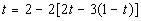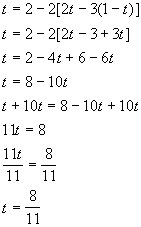*Distribute the inner most (   ) *Distribute the outer grouping [  ]   *Get all t terms on one side   *Inverse of mult. by 11 is div. both sides by 11

 If you put 8/11 back in for t in the original problem you will see that 8/11 is the solution we are looking for. (return to problem 1c)Answer/Discussion to 1d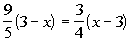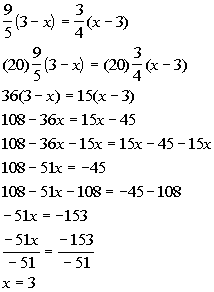*Mult. both sides by the LCD of 20   *Use dist. property to remove (  ) *Get all x terms on one side *Inverse of add. 108 is sub. 108   *Inverse of mult. by -51 is div.  by -51

 If you put 3 back in for x in the original problem you will see that 3 is the solution we are looking for. (return to problem 1d)Answer/Discussion to 1e 7(x - 3) = 7x + 2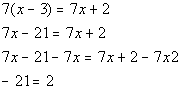*Use dist. property to remove (  )  *Get all x terms on one side

 Since the variable dropped out AND we ended up with a false statement, the answer is no solution. (return to problem 1e)

Last revised on July 1, 2011 by Kim Seward.
All contents copyright (C) 2002 - 2011, WTAMU and Kim Seward. All rights reserved.